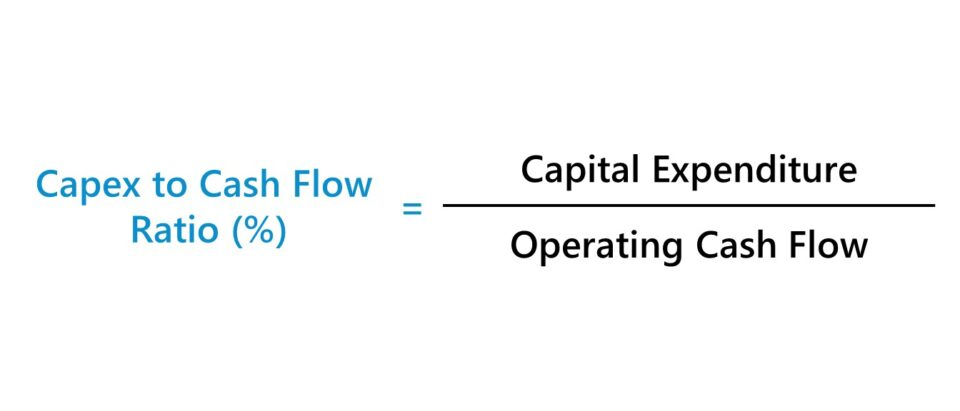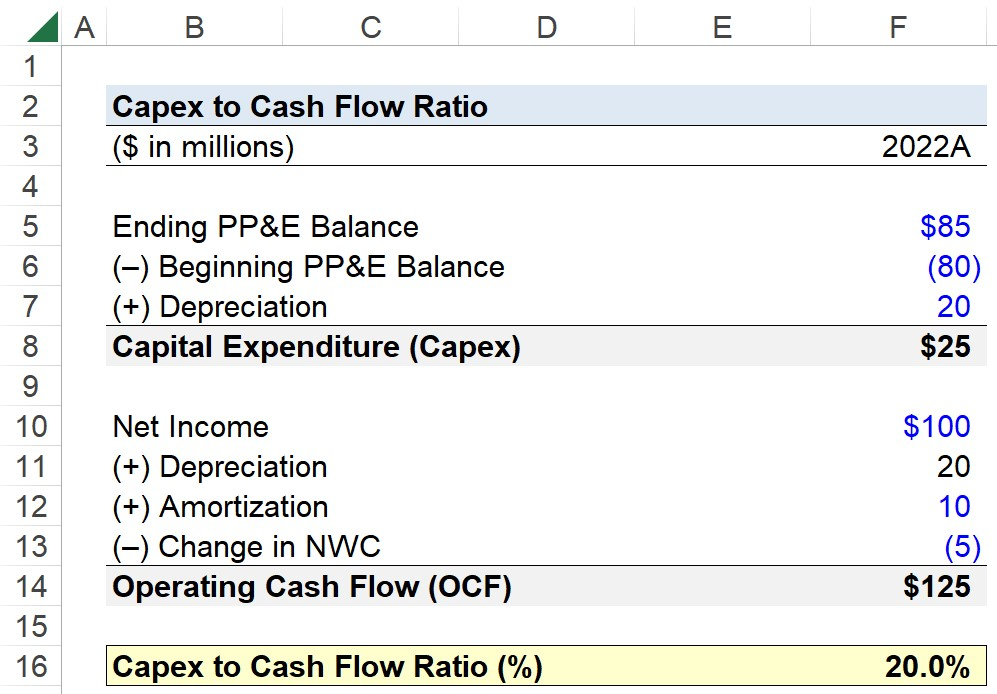Welcome to Wall Street Prep! Use code at checkout for 15% off.# Capex to Cash Flow Ratio

Guide to Understanding the Capex to Cash Flow Ratio (%)## How to Calculate Capex to Cash Flow Ratio?

The capex to cash flow ratio is a method to measure the efficiency at which a company utilizes its operating cash flow (OCF) towards the purchase of fixed assets (PP&E).

The calculation of the capex to cash flow ratio involves comparing a company’s capital expenditures to its operating cash flow (OCF).

• Capital Expenditures (Capex) → The term capital expenditures (Capex) refers to spending used towards the purchase of fixed assets (PP&E). The former describes “maintenance capex”, whereas the latter refers to “growth capex.” While the spending categorized as growth capex is more discretionary, maintenance capex refers to the minimum spending required to support ongoing operations (e.g. the replacement of equipment).
• Operating Cash Flow (OCF) → The operating cash flow (OCF) metric, or “cash flow from operations”, is the amount of cash generated by the core operating activities of a company over a specified period.

The allocation of the remaining cash flow from operations (CFO) is up to management discretion. For instance, the cash can be issued to common shareholders in the form of dividends or used for stock buybacks, capital expenditures, business acquisitions, and more.

All else being equal, a company that spends less on capital expenditures per dollar of operating cash flow, yet generates the same (or more) revenue than its industry peers is more efficient at utilizing its fixed asset base.

The steps to calculate the capex to cash flow ratio (%) consist of a three-step process:

1. Total Capex Spend → The capital expenditures of a company can be found on the cash flow statement (CFS) in the cash from investing section.
2. Operating Cash Flow (OCF) → The starting point for the calculation of operating cash flow (OCF) is net income—i.e. the accrual-based profitability metric inclusive of all operating and non-operating costs—and then non-cash items (e.g. the add-back on depreciation and amortization) and the change in net working capital (NWC) must be reconciled.
3. Capex to Cash Flow Ratio (%) → The numerator, capex, is divided by the denominator, operating cash flow (OCF), which results in the capex to cash flow ratio. The ratio must then be multiplied by 100 to convert it into percent form.

## Capex to Cash Flow Ratio Formula

The formula to calculate the capex to cash flow ratio is as follows.

Capex to Cash Flow Ratio (%) = Capital Expenditures ÷ Operating Cash Flow (OCF)

Where:

• Operating Cash Flow (OCF) = Net Income + Depreciation and Amortization (D&A) – Change in Net Working Capital (NWC)
• Capital Expenditure (Capex) = (Ending PP&E – Beginning PP&E) + Depreciation

## Capex to Cash Flow Ratio Calculator

We’ll now move on to a modeling exercise, which you can access by filling out the form below.Submitting...

## 1. Operating Assumptions

Suppose you’re tasked with calculating the capex to cash flow ratio of a company given the following operating assumptions for fiscal year 2022.

Fixed Asset (PP&E) Assumptions

• Beginning PP&E = \$80 million
• Ending PP&E = \$85 million
• Depreciation = \$20 million

Operating Assumptions

• Net Income = \$100 million
• Depreciation = \$20 million
• Amortization = \$10 million
• (Increase) / Decrease in Net Working Capital (NWC) = (\$5 million)

First, we’ll determine our company’s capital expenditures (Capex) by deducting the beginning PP&E balance from the ending PP&E balance—with the end-of-period balance entered as a negative number—before adding back the non-cash depreciation expense.

• Capital Expenditure (Capex) = \$85 million – \$80 million + \$20 million = \$25 million

Next, we’ll calculate the company’s operating cash flow (OCF) in the same period by taking its net income and reconciling it for non-cash items (e.g. D&A) and the change in net working capital (NWC).

• Operating Cash Flow (OCF) = \$100 million + \$20 million + \$10 million – \$5 million = \$125 million

## 2. Capex to Cash Flow Ratio Calculation Example (%)

Lastly, we’ll enter the operating cash flow (OCF) and capital expenditures (Capex) into the formula from earlier to arrive at a capex to cash flow ratio of 20.0%, which is the percentage of the company’s operating cash flow allocated towards its capex spending.

The implied proportion of operating cash spent on capex (%) is a standardized metric and can thereby be compared to the company’s historical capex spending and that of its industry peers to gauge its relative capex efficiency.

• Capex to Cash Flow Ratio (%) = \$25 million ÷ \$125 million = 0.20, or 20%Step-by-Step Online Course

### Everything You Need To Master Financial Modeling

Enroll in The Premium Package: Learn Financial Statement Modeling, DCF, M&A, LBO and Comps. The same training program used at top investment banks.